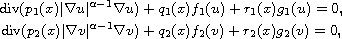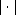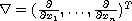Electron. J. Diff. Equ., Vol. 2014 (2014), No. 214, pp. 1-10.

### Sturm-Picone type theorems for second-order nonlinear elliptic differential equations Aydin Tiryaki

Abstract:wheredenotes the Euclidean length and(the superscript T denotes the transpose). Our results include some earlier results and generalize to n-dimensions well-known comparison theorems given by Sturm, Picone and Leighton ]26.37] which play a key role in the qualitative behavior of solutions. By using generalization of n dimensional Leigton's comparison theorem, an oscillation result is given as an application.Aydin Tiryaki Department of Mathematics and Computer Sciences Faculty of Arts and Sciences, Izmir University 35350 Uckuyular, Izmir, Turkey email: aydin.tiryaki@izmir.edu.tr